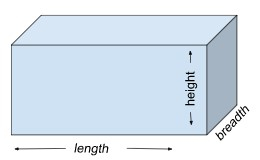# Golang program to Calculate the Volume, Diagonal and Area of a Cuboid?

In this tutorial, we will discuss the approach to calculating the volume, diagonal and area of a cuboid using its length, breadth and height.

But before writing its Golang code, let’s briefly discuss the cuboid and its volume, diagonal and area.

## Cuboid

A cuboid is a three−dimensional figure with six faces and twelve edges. The length, breadth, and height of a cuboid are different unlike a cube whose all sides are equal. A rectangular box is a good example of a cuboid.## Volume of a Cuboid

The amount of space a cuboid occupies is termed its volume. Calculating the volume of a cuboid can be beneficial in situations where we want to know how much air can be accumulated in a room.

Volume = l * b * h


## Diagonal of a cuboid

The diagonal of a cuboid can be defined as the line segment joining two opposite vertices of the cuboid.

$$\mathrm{Diagonal\: = \:\sqrt({l^2 + b^2 +h^2})}$$

## Area of a cuboid

The total area occupied by the cuboid is known as the area of the cuboid.

Area = 2 * (l * h + l * b + h * b)


### Example

• Length = 10, Breadth = 3, Height = 5

Volume of cuboid = 150

Diagonal of cuboid = 11.575836902790225

Area of cuboid = 190

### Explanation

$\mathrm{Volume\: of\: cuboid \:= \:l * b * h}$

$\mathrm{= \:10 * 3 * 5}$

$\mathrm{=\: 150}$

$\mathrm{Diagonal\: of\: cuboid\: = \:\sqrt({l^2 + b^2 +h^2})}$

$\mathrm{\:\sqrt({l0^2 +3^2 +5^2})}$

$\mathrm{= 11.575836902790225}$

$\mathrm{Area\: of\: cuboid\: = \:2 * (l * h + l * b + h * b)}$

$\mathrm{= \:2 * (10 * 5 + 10 * 3 + 5 * 3)}$

$\mathrm{= \:190}$

## Calculating the Volume, Diagonal and Area of a Cuboid

• Step 1 − Declare three variables for storing the length ‘l’, breadth ‘b’, and height ‘h’ of the cuboid.

• Step 2 − Declare three variables for storing the volume of the cuboid− ‘volume’, diagonal ‘diag’ and area of the cuboid− ‘area’ and initialize all the three variables with the value 0.

• Step 3 − Calculate the volume using the formula− Volume = l * b * h, and store it in the ‘volume’ variable in the function calculateVolumeOfCuboid().

• Step 4 − Calculate the diagonal using the formula− Diagonal = √(l2 + b2 +h2), and store it in the ‘diag’ variable in the function calculateDiagonalOfCuboid().

• Step 5 − Calculate the area using the formula− Area = 2 * (l * h + l * b + h * b), and store it in the ‘area’ variable in the function calculateAreaOfCuboid().

• Step 6 − Print the calculated volume, diagonal and area of the cuboid, i.e, the value stored in the ‘volume’, ‘diag’ and ‘area’ variables.

### Example

package main
import (
"fmt"
"math"
)
func calculateVolumeOfCuboid(l, b, h float64) float64 {
var volume float64 = 0
volume = l * b * h
return volume
}
func calculateDiagonalOfCuboid(l, b, h float64) float64 {
var diag float64 = 0
diag = math.Sqrt((l * l) + (b * b) + (h * h))
return diag
}
func calculateAreaOfCuboid(l, b, h float64) float64 {
var area float64 = 0
area = 2 * ((l * h) + (l * b) + (h * b))
return area
}
func main() {
var l, b, h float64 = 10, 3, 5
var volume, diag, area float64
fmt.Println("Program to calculate the volume, diagonal and area of the Cuboid \n")
volume = calculateVolumeOfCuboid(l, b, h)
diag = calculateDiagonalOfCuboid(l, b, h)
area = calculateAreaOfCuboid(l, b, h)

fmt.Println("Length of the cuboid: ", l)
fmt.Println("Breadth of the cuboid: ", b)
fmt.Println("Height of the cuboid: ", h)
fmt.Println("Therefore, Volume of cuboid: ", volume)
fmt.Println("Diagonal of cuboid: ", diag)
fmt.Println("Area of cuboid: ", area)
}


### Output

Program to calculate the volume, diagonal and area of the Cuboid

Length of the cuboid:  10
Height of the cuboid:  5
Therefore, Volume of cuboid:  150
Diagonal of cuboid:  11.575836902790225
Area of cuboid:  190


### Description of Code

• calculateVolumeOfCuboid(l, b, h float64) float64 − This function will calculate the volume of the cuboid. It has a return type of float64.

• diag = math.Sqrt((l * l) + (b * b) + (h * h)) − Using this formula, we are calculating the diagonal. We are using the Sqrt function from the math package to do the square root.

## Conclusion

This is all about calculating the volume, diagonal and area of a cuboid using Go programming. We have also maintained code modularity by using separate functions for calculating the area, diagonal and volume which also increases the code reusability. You can explore more about Golang programming using these tutorials.